# LogBERT: log file anomaly detection using BERT: An Explainer

Authored by Syed Abdul and Raja Rajendran

# 1.1 Introduction

This is the first article in a two part series:

2. Training and inferencing of LogBERT, using an ML pipeline running on Infinstor MLOps platform (to be published shortly)

LogBERT [1,2] is a self-supervised approach towards log anomaly detection based on Bidirectional Encoder Representations from Transformers (BERT). The objective is to detect anomalies in logs generated by online systems, by learning the underlying patterns of normal log sequences, and to detect if there are any deviations from these normal log patterns.

Earlier techniques to detect log anomalies were rules-based and machine learning based,which are discussed below.

• Detecting log lines that are anomalous using keywords or regular expressions: the downside of this approach is that it can detect if a single line is an anomaly or not but cannot handle scenarios where the single log line is not an anomaly but the sequence of log messages is an anomaly .
• Another approach is to write rules to detect anomalies in the log sequence: the downside is that previously unseen anomalies cannot be detected (for which rules are yet to be created). To accommodate such unseen anomalies, the rules have to be updated constantly to handle them.

Machine Learning Based approaches

• A classic binary classification problem where the model is trained to classify logs as “normal” and “anomaly” using previous examples of logs of normal and anomaly logs. This approach is not used in practice due to very high data imbalance between normal and anomalous logs.
• Widely used Traditional Machine Learning are unsupervised learning algorithms like Principal Component Analysis (PCA) or one-class SVM, where the logs are clustered based on normal and anomalous categories, the downside is it’s very hard to capture the temporal information of log messages.

Deep Learning based Approaches:

• Recurrent Neural Networks (RNNs) are widely used for log anomaly detection since they are able to model sequential data. These are good for sequences of logs, and can detect anomalies which are part of sequence, but the drawback is the context information from both the left and right context, which is crucial to observe complete context not just from previous log messages. In addition, there is also the limitation of context/memory held when processing long log sequences.

To tackle the above-mentioned problems in traditional and RNN based approaches, LogBERT [1,2], a Transformer based approach, is introduced. This approach relies on the success of BERT in modeling sequential data. By leveraging BERT, the pattern of normal log sequences are learned during training. The self-attention mechanism of BERT is used to convert each log line template (log key) to a contextual embedding, which captures information about the association of this log key to the other log keys that surround it in a log sequence.

# 1.2 Training Framework

LogBERT is trained using self-supervised training, to create a language model solely using the normal logs from the dataset, to capture normal log sequence patterns.

1. The examples below use the BGL dataset , where we first extract the log keys (string templates) from log messages via a log parser. Each log line in the dataset is passed through the log parser to obtain this log key (log template).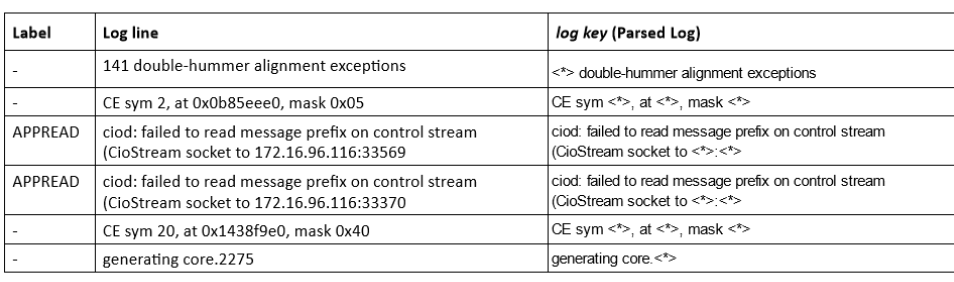Table 1: Mapping from a ‘log line’ to its ‘log key’ (Events)

Here the log line with Label ‘-’ indicates an anomalous log line. The log parsers available with LogBERT repo are ‘Drain’ and ‘Spell’.

2. Each parsed log key is then given a unique event id, which is used as the vocabulary for training of BERT. While creating this vocabulary, we take only those events(log keys) which occur more than a specified threshold value. In our case, this threshold value is 1. So, events(log keys) which are seen in the input dataset at least twice are included in the vocabulary, while the others being dropped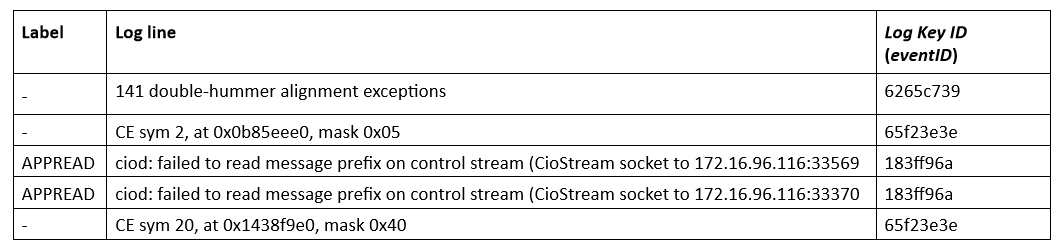Table 2 Mapping from a ‘log line’ to its ‘log key ID’ or ‘eventID’.

3. We now convert the log lines to log sequences. We can do this in a few different ways:

a. sliding window technique:

• using a sliding window with a window size, to create log sequences, where all logs in a time window ( say 5 minutes) are structured as a log sequence.
• the step_size indicates how much the sliding window slides in each step (say 1 minute)

b. fixed time window technique

c. log attribute based approach

d. number of log lines approach

Shown below are some log sequences generated using a sliding window.

4. Each log sequence is now a sequence of log keys {k¹, k², k³, …, kᵗ, …, kᵀ⁻¹, k} as shown in the picture above. We also add a unique special token kᵈᶦˢᵗ, at the beginning of each log sequence, which is used as Distance token. This token is used to calculate the distance between this log sequence and the center (the center is computed using all log sequences in the input), after passing through BERT.

5. We now have an input dataset of log sequences where the label indicates if the ‘log sequence’ is normal ( 0 ) or anomalous (1).

5. Next, create a randomly generated matrix E ∈ Rᴷ*ᵈ ( see Figure 1. Overview of LogBERT above ) which represents the log key embedding (like word embedding) where each row in the matrix represents embedding of each key in the vocabulary.

• d’ here is the dimension of each log key embedding.
• |K| indicates a set of log keys extracted from log messages.

Along with this embedding, we also create positional embedding T ∈ Rᵀ*ᵈ , generated using a sinusoidal function, to encode the position information of log keys in log sequence ( see Figure 1. Overview of LogBERT above ) .

LogBERT represents each log key kᵗⱼ as an input representation xᵗⱼ ( see Figure 1. Overview of LogBERT above ) , where the input representation xᵗⱼ is a summation of a log key embedding(eᵗⱼ)and a position embedding(tᵗⱼ).

6. The above computed input representation {xᵈᶦˢᵗ, x¹, x², x³, …, xᵗ, …, xᵀ⁻¹, x} is fed as an input to logBERT’s Transformer Encoder. xᵈᶦˢᵗ is the input representation of the distance token added to the beginning of each log sequence. This input is passed through the Transformers self-attention mechanism with stacked transformers layers similar to BERT.

When feeding the input representation to the transformer, we mask a ratio of tokens randomly as part of the training objective. The output of this transformer encoder is the contextualized embedding {hᵈᶦˢᵗ, h¹, h², h³, …, hᵗ, …, hᵀ⁻¹, h}, one for each log key in the log sequence ( see Figure 1. Overview of LogBERT above ) .

7. The computed contextual embedding hᵐᵃˢᵏ for masked tokens are passed to a fully connected layer and then to softmax function, to get the probability distribution of the token over the vocabulary ( see Figure 1. Overview of LogBERT above ). This distribution is used to predict the most suitable token in place of MASKed token.

where W꜀ and b꜀ are trainable parameters.

We adopt the cross entropy loss as the loss function for masked log key prediction (MLKP) task, which is defined as:

where,

• yʲₘₐₛₖ indicates the predicted probability distribution of the masked log key over the vocabulary, in the jᵗʰ log sequence
• and M is the total number of masked tokens in the log sequence j.

So the objective is to learn to predict the MASKed tokens in the log sequence, where higher the probability of the actual log key in the predicted probability distribution yʲₘₐₛₖ , then lower is the loss value Lₘₗₖₚ.

Another objective function used is the Volume of Hypersphere Minimization, where the hᵈᶦˢᵗ is used to reduce the distance between the hᵈᶦˢᵗ and the center c ( see Figure 1. Overview of LogBERT above ).

where c is the center representation of normal log sequence in the training dataset, c = Mean(hᵈᶦˢᵗ).

During the training phase, the model learns/adjusts its weights so as to minimize the above loss functions.

# 1.3 Inferencing Pipeline

1. Once the training is done, the logBERT model understands the normal log patterns since it’s trained on normal logs, and can achieve high prediction accuracy on predicting the masked tokens(masked log lines) in the log sequence.

2. Just like training, we mask the tokens randomly in the sequence, and run through the model to predict the MASK tokens in the sequence based on the Softmax given probability distribution to get predictions.

3. Once the predictions of MASK tokens are achieved, for each MASK token prediction we take top-g predictions. In other sense, these top-g predictions are expected logs to be there if normal patterns.

4. We then check if the actual log from the sequence which was masked, is present in the top-g candidates, if present then we treat the key as normal and if the actual log is not present in the prediction list of that MASK token, then the log is considered to be anomalous.

5. For a given sequence, if there are more than r anomalous logs then we consider the log sequence to be anomalous. The g and r are both hyper-parameters values and can be tuned based on the dataset.

# 1.4 LogBERT Google Colab Notebook for Training and Prediction

See https://colab.research.google.com/drive/1_msJIS-BCMKPrPIlsZSJfUIZttgfoVry?usp=sharing for Google colab notebook for training and prediction using logBERT.

# 1.5 Summary

LogBERT uses a novel approach of creating a language model for log sequences (using BERT) using normal log sequences. The training is done by masking random log keys in the log sequence and training the model to predict the masked log keys correctly. The training phase uses two loss functions described above to learn the model weights.

During prediction, LogBERT will be able to predict masked log keys in a log sequence. if the actual(true) log key (which was masked) is different from the predicted log key, and if the number of mismatches exceeds the hyperparameter r, then the log sequence is marked as an anomalous log sequence.

# 1.6 References

1. Guo, Haixuan. “logBERT github repo.” https://github.com/HelenGuohx/logbert.

2. Guo, Haixuan, et al. “LogBERT: Log Anomaly Detection via BERT.” 2021, https://arxiv.org/abs/2103.04475.

3.Yinglung Liang, et al, . “Filtering failure logs for a BlueGene/l”. In 2005 International Conference on Dependable Systems and Networks
(DSN’05). IEEE, 476–485.

4. USENIX, BGL Dataset. https://www.usenix.org/cfdr-data#hpc4

--

--

--

## Key concepts of neural network optimization## Support Vector Machine (SVM)## Training T5 model in just 3 lines of Code with ONNX Inference## Spello — The Spell Correction Library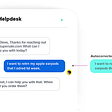## Speech Recognition on Google Speech Commands — By Basic LSTMCells## Making a Deep Learning Chandler Bing AI (from f.r.i.e.n.d.s)Part-1## The right way of using SMOTE with Cross-validation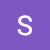## Syed Abdul

Data Scientist @ infinstor.com

## Analyzing conversations by using transformers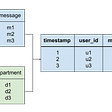## DocArray → A type agnostic data structure!## Introducing LM-Debugger## NLP-Day 24: Know Your Place. Positional Encoding In Transformers (Part 2)